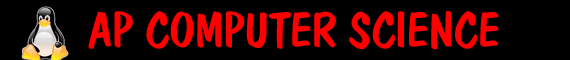Quiz 27b Recursion - really hard - you have 10 submits   id:     1. `````` public void mystery (int x) { if (x <= 0) return; else { System.out.print( x + ""); mystery( x - 3); } } `````` What does mystery(5) print?   2. ``````public void mystery (int x) { if (x <= 0) return; else { mystery (x - 2); System.out.print(x + "");} } `````` What does mystery(5) print? 3. ``````public int mystery (int x) { if (x <= 0) return 1; else return x * mystery(x- 2); } `````` What does mystery(5) return? 4. ``````public int mystery (int x) { if (x<= 10) return x; else return ((x / 10) + mystery(x/10)); } `````` What does mystery(541) return? 5. `````` public int mystery(int x) { if (x == 1) return 2; else return mystery(x - 1)*2; } `````` What does mystery(5) return? 6. ``````public int mystery(int x) { if (x <= 0) return 0; else return x + mystery( x-3) + mystery ( x-5); } `````` What does mystery(8) return? 7. Which one could lead to an infinite loop?